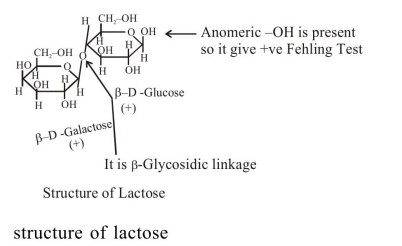# Which one of the following statements not true ?

Question:

Which one of the following statements not true ?

1. Lactose contains $\alpha-\mathrm{glycosidic}$ linkage between $C_{1}$ of galactose and $C_{4}$ of glucose.

2. Lactose $\left(\mathrm{C}_{11} \mathrm{H}_{22} \mathrm{O}_{11}\right)$ is a disaccharide and it contains 8 hydroxyl groups.

3. On acid hydrolysis, lactose gives one molecule of $\mathrm{D}(+)$-glucose and one molecule of $\mathrm{D}(+)$-galactose.

4. Lactose is a reducing sugar and it gives Fehling's test.

Correct Option: 1

Solution: